Foxtable(狐表)用户栏目专家坐堂 → [求助]for each语句

共有65人关注过本帖树形打印复制链接

# 主题：[求助]for each语句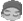l1q2lq
1楼 | 信息 | 搜索 | 邮箱 | 主页 | UC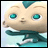加好友发短信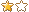[求助]for each语句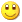Post By：2020/5/24 10:47:00 [只看该作者]

 老师 有一段代码如下想实现如果库存表中有只要一行缺货，就无法出库但是实际效果是：如果001有货，002缺货，003有货，就会把001出库，002,003停止执行代码需要怎么修改For Each r1 As Row In Tables("库存表")    If r1("库存状态") = "缺货" Then        MessageBox.Show("库存不足!")        Exit For    Else        Dim  r2 As Row = Tables("出库表").AddNew        r2("零件号") = r1("零件号")        r2("出库数量") = r1("发货数量")Next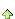DataWorker
2楼 | 信息 | 搜索 | 邮箱 | 主页 | UC加好友发短信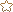Post By：2020/5/24 11:16:00 [只看该作者]

 Dim i As Integer = 0        For Each r1 As Row In Tables("库存表")            If r1("库存状态") = "缺货" Then                i = i + 1            End If        Next        If i = 0 Then            For Each r1 As Row In Tables("库存表")                Dim r2 As Row = Tables("出库表").AddNew                r2("零件号") = r1("零件号")                r2("出库数量") = r1("发货数量")            Next        Else            MessageBox.Show("库存不足!")        End If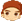3楼 | 信息 | 搜索 | 邮箱 | 主页 | UC加好友发短信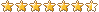Post By：2020/5/24 21:46:00 [只看该作者]

 If Tables("库存表").Compute("count(库存状态)","库存状态='缺货'") > 0 thenMessageBox.Show("库存不足!")else            For Each r1 As Row In Tables("库存表")                Dim r2 As Row = Tables("出库表").AddNew                r2("零件号") = r1("零件号")                r2("出库数量") = r1("发货数量")            Nextendif